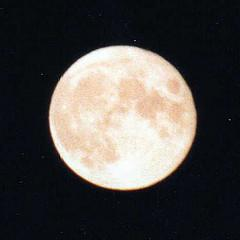# Michael Jackson

Commenting is disabled.

# Post ContentMichael Joseph Jackson, the seventh of nine children of the Jackson family, was born August 29, 1958 in Indiana. Happy June 25 2009goda a performer who was at his home in Los Angeles, a sudden cardiac arrest. This happened about noon local time. According to the method of additional numbers AF Alexandrov, each date has a second set of numbers. Below is a calculation of the second series of numbers to dates of birth of Michael Jackson. We write out the number line date Birth: 29 August 1958, 1.

We calculate the first number. To calculate the first number, add up all the digits of the numerical series date of birth. 2 +9 +8 +1 +9 +5 +8 = 42, the first number – 42 (1). 2. We calculate the second number. To calculate the second number, add up the numbers, of which is the first number (1).

4 +2 = 6, the second number – 11 (2). 3. We calculate the third. To calculate the third number to be subtracted from the first number (1) the first digit of the entire series (in our example, figure 2), multiplied by a constant factor – 2 (two). 42-2×2 = 42-4 = 38, third number – 38 (3). 4. We calculate the fourth number. To calculate the fourth day, add up the numbers that make up the third number (3). 3 +8 = 11, the fourth number – 11. (4) Record the number of second-line 42 6 38 11. The presence of combinations of: 20.2, 15.6, 24.6, 18.9, 27.9, 36.9, 6.6, 9.9, and additional numbers of the birth of the second and fourth days of the projected additional day should inspire anyone who refers to the events of that day very carefully.